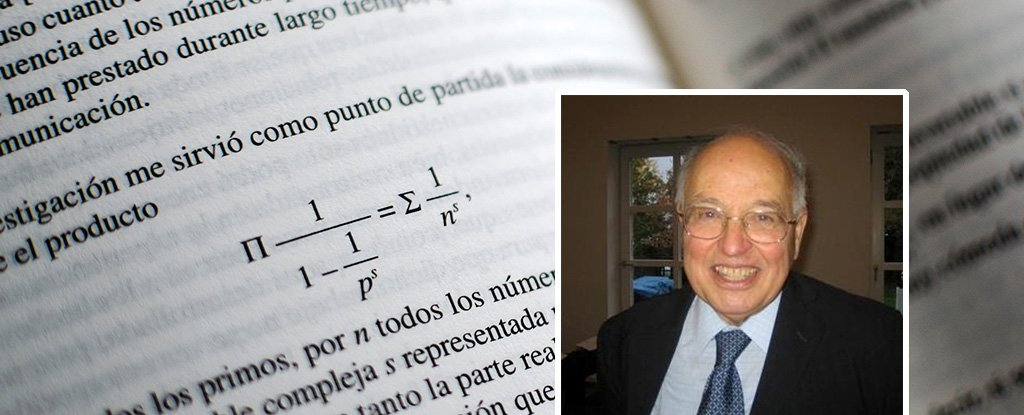Humans# Top mathematician says he’s solved a 160-year-old maths problem worth \$1 million

One of the world’s most renowned mathematicians showed how he solved the 160-year-old Riemann hypothesis at a lecture on Monday – and he will be awarded US\$1 million if his solution is confirmed.

Sir Michael Atiyah, who has won the two biggest prizes in mathematics – the Fields Medal and Abel Prize – took the stage at the Heidelberg Laureate Forum in Germany on Monday to present his work.

To solve the hypothesis you need to find a way to predict the occurrence of every prime number, even though primes have historically been regarded as randomly distributed.

Aityah’s solution will need to be checked by other mathematicians and then published before it is fully accepted and he can claim the prize from the Clay Mathematics Institute of Cambridge.

The Riemann hypothesis is one of seven unsolved “Millennium Prizes” from CMI, each worth US\$1 million to the person who solves it.

What is the Riemann hypothesis, and how did Atiyah solve it?

The Riemann hypothesis was first posited by Bernhard Riemann in 1859.

It attempts to answer an old question about prime numbers (numbers that divide only by themselves and 1.) The hypothesis states that the distribution of primes is not random, but might follow a pattern described by an equation called the Riemann zeta function.

10,000,000,000,000 prime numbers have been checked and are consistent with the equation, but there is no proof that all primes follow the pattern.

So, the US\$1 million prize goes to someone who can prove that the equation applies to all prime numbers. And Atiyah, using a “radically new approach” to the hypothesis, according to his explanation of his solution, thinks he has done it.

Markus Pössel‏, an astrophysicist in Heidelberg, Germany, live-tweeted Atiyah’s lecture and helped clarify the mathematician’s process:

Atiyah said in the lecture that he used work from John von Neumann and Friedrich Hirzebruch to help him on his way to solving the problem.

Mathematician Keith Devlin wrote in 1998: “Ask any professional mathematician what the single most important open problem in the entire field is, and you are almost certain to receive the answer ‘the Riemann hypothesis.'”

Atiyah has also served as president of the London Mathematical Society, the Royal Society, and the Royal Society of Edinburgh.

CMI declined to comment when reached by Insider.